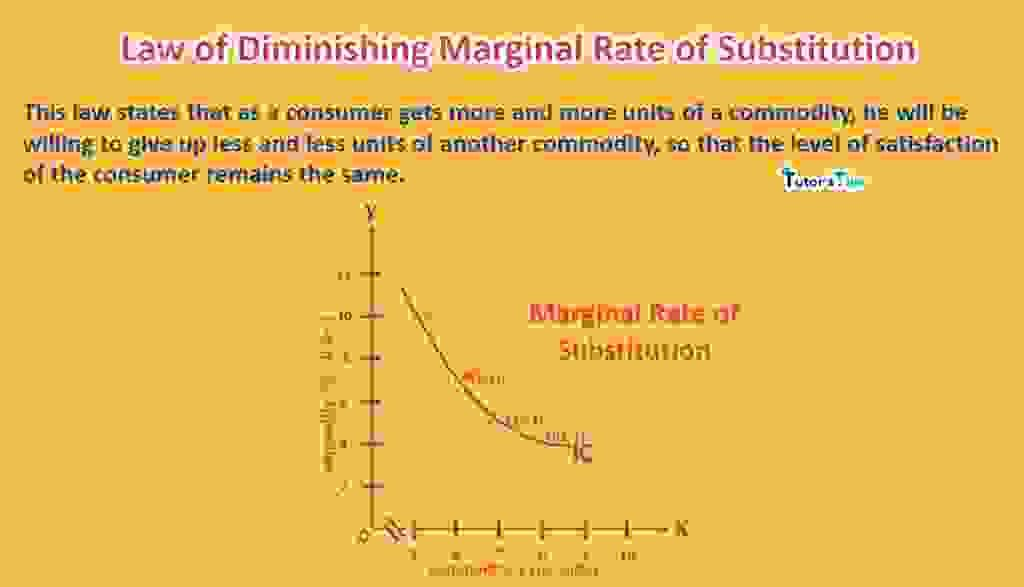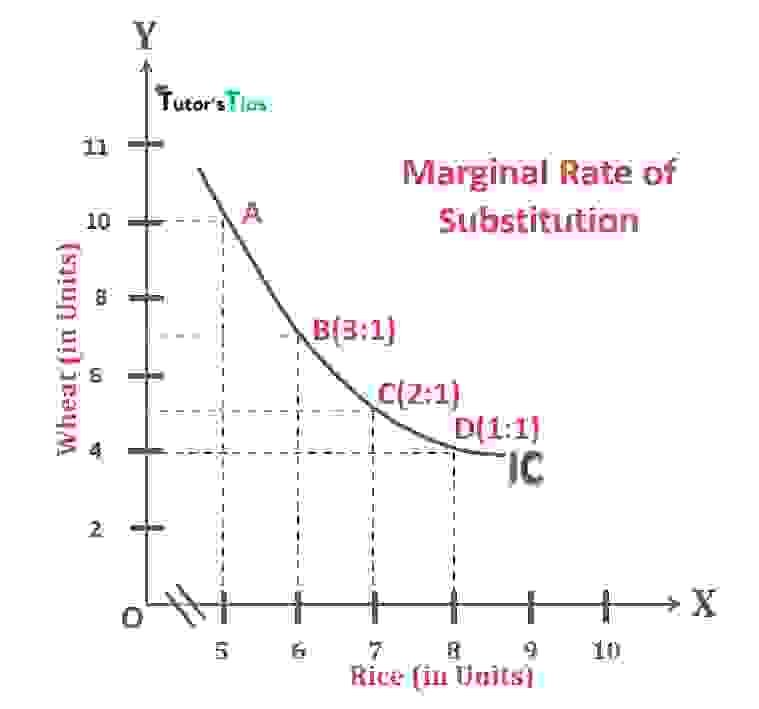# Law of Diminishing Marginal Rate of SubstitutionThe concept of indifference curve analysis is based on the law of diminishing marginal rate of substitution. It states, with more consumption of one commodity, the marginal rate of substitution declines.

## What is the Marginal Rate of Substitution:

As indifference curve explains that when a customer gets one more unit of a commodity, he has to sacrifice some units of another commodity to retain at the same level of satisfaction. In other words, in exchange for the satisfaction obtained from an additional unit of commodity-1, the consumer has to give up that many units of commodity-2 whose satisfaction is equal to additional satisfaction obtained from an additional unit of commodity-1.

Subscribe our Youtube Channel

Utility gained from more unit of commodity-1= Utility lost of commodity-2

Thus, the Marginal Rate of Substitution is the rate at which consumer can substitute one commodity for another without changing the level of satisfaction. It indicates the slope of indifference curves.

## Definition Marginal Rate of Substitution:

In the words of Prof. Bilas,

“The marginal rate of substitution of X for Y is defined as the amount of Y, the consumer is willing to give up to get one more unit of X and maintain the same level of satisfaction.”

 MRSXY = Loss of Y = (-) ΔY Gain of X ΔX

Here,

MRSXY  represents the Marginal Rate of Substitution of X for Y

ΔY represents the change in Y

ΔX represents the change in X

In short, the marginal rate of substitution is the ratio of the amount of Y that must be sacrificed per unit of X gained if the consumer is to remain at the same level of satisfaction. Since the effect of change in Y with respect to X is opposite. Therefore, the ratio is negative.

## Law of Diminishing Marginal Rate of Substitution :

This law states that as a consumer gets more and more unit of a commodity, he will be willing to give up less and fewer units of another commodity so that the level of satisfaction of the consumer remains the same. In other words, the marginal rate of substitution of X for Y will go on diminishing.

According to Ferguson,

“The law of diminishing marginal rate of substitution states that as X is substituted for Y soo as to leave the consumer on the same indifference curve, the marginal rate of substitution of X for Y diminishes.”

### Explanation with Illustration:

 Combination of Wheat and Rice Rice(in units) Wheat(in units) MRS A 5 10 B 6 7 3:1 C 7 5 2:1 D 8 4 1:1

In the above table, it is clearly shown that the consumer will give up 3 units of wheat for getting an additional unit of rice as the consumption of wheat declines to 7 units from 10 units with an increase in consumption of just one more unit of rice. Similarly, as consumer consumes one more unit of rice, it declines the consumption of wheat from 7 to 5 units, sacrificing 2 units of wheat for one more unit of rice. Likewise, an increase in unit consumption of rice results in the sacrifice of 1 unit of wheat. Thus, the marginal rate of substitution of rice for wheat goes on diminishing.

### Graphical Representation:Graphical Representation of the Marginal Rate of Substitution

In fig, X-axis represents the quantity of rice and Y-axis represents the quantity of wheat. The graph, clearly shows that when a consumer moves from A to B, he has to give up 3 units of wheat to obtain one additional unit of rice. Therefore, the consumer’s marginal rate of substitution of rice for wheat is said to be 3:1.

When he moves from B to C, he gives up only 2 units of wheat to get an additional unit of rice. Here, the marginal rate of substitution of rice for wheat is 2:1. Thus, it is cleared that as the consumer increases the consumption of rice, then for getting every additional unit of it, he gives up less and fewer units of wheat i.e. 3:1,2:1 and 1:1 respectively. Hence, it is known as the Marginal rate of substitution and the law relating to it is the law of Diminishing Marginal rate of substitution.

## Comparison of Laws: –

Law of Diminishing Marginal Utility and Law of Diminishing Marginal Rate of Substitution:

According to Prof.Hicks, the law of diminishing marginal rate of substitution explains this tendency of consumer behaviour with far fewer assumptions than the law of diminishing marginal utility. Consequently, the law of diminishing marginal rate of substitution  is more realistic than the law of diminishing marginal utility due to the following reasons:

### 1) Measurement of Utility:

Law of diminishing marginal utility is based on the assumption that utility can be measured in cardinal numbers. But, in the law of diminishing marginal rate of substitution, there is no need for measuring the utility. Therefore, this law is more realistic.

### 2) Interdependent commodities involved:

The law of diminishing utility is based on the assumption that only one commodity is consumed by the consumer and the utility depends upon the availability of that commodity only. But, in the law of diminishing marginal rate of substitution, there is no need for such an assumption. Whether this law considers the effect of utilities of related goods on each other. Thus, it recognizes the interdependency of commodities on each other.

### 3) Marginal Utility of Money:

The law of diminishing marginal utility assumes that the marginal utility of money remains constant, which is unrealistic. On the other hand, the law of diminishing marginal rate of substitution ignores such unrealistic assumption.

Thanks Please share with your friends

Comment if you have any question.

References:

Introductory Microeconomics – Class 11 – CBSE (2020-21)